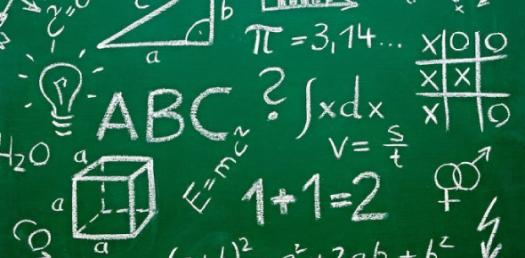# Simple Math GK Trivia

15 Questions | Total Attempts: 148SettingsCreate your own QuizHere are some more questions about general knowledge math!

• 1.
Which of these sets is not a fractal?
• A.

Dragon Curve

• B.

Mandelbrot Set

• C.

Sierpinski Triangle

• D.

Parabola

• 2.
What is the inverse of f(x) = 3x + 5?
• A.

-3x + 5

• B.

5x + 3

• C.

(1/3)x - 5/3

• D.

-3x - 5

• 3.
What is the equation of a line with a slope of 5/3 and a y-intercept of 6?
• A.

5x + 3y = 18

• B.

5x + 3y = -18

• C.

5x - 3y = -18

• D.

5x - 3y = 18

• 4.
Which of these shows an absolute value function?
• A.

F(y) = x

• B.

F(y) = 1

• C.

F(x) = 1

• D.

F(x) = x

• 5.
Which of these shows the identity function?
• A.

F(y) = x

• B.

F(y) = 1

• C.

F(x) = 1

• D.

F(x) = x

• 6.
How many degrees are there in a triangle?
• A.

360

• B.

180

• C.

90

• D.

720

• 7.
What is the square root of 400?
• A.

1600

• B.

100

• C.

40

• D.

20

• 8.
What is the approximate value of pi plus 2 and one half?
• A.

3.84

• B.

5.64

• C.

3.24

• D.

4.55

• 9.
How many years are in a millennium?
• A.

10,000

• B.

10

• C.

1,000

• D.

100

• 10.
Which is the smallest?
• A.

3 Cm

• B.

3 Mm

• C.

3 Inches

• D.

3 Meters

• 11.
Which "Father of Modern Mathematics" is known for the statement "I think, therefore I am"?
• A.

Leonhard Euler

• B.

Evariste Galois

• C.

Marin Mersenne

• D.

Rene Descartes

• 12.
On a test if you get 18 right out of 20, what percent did you get correct?
• A.

90%

• B.

92%

• C.

80%

• D.

75%

• 13.
Our Pizza had 8 slices, we ate 6, what fraction was left?
• A.

3-Jan

• B.

5-Jan

• C.

4-Jan

• D.

2-Jan

• 14.
What term means the territory taken up by a surface?
• A.

Volume

• B.

Array

• C.

Base Number

• D.

Area

• 15.
What number comes next in this sequence: 4,16,5,25,6,..?
• A.

36

• B.

50

• C.

24

• D.

7

Related TopicsBack to top
×

Wait!
Here's an interesting quiz for you.